$$\newcommand{\id}{\mathrm{id}}$$ $$\newcommand{\Span}{\mathrm{span}}$$ $$\newcommand{\kernel}{\mathrm{null}\,}$$ $$\newcommand{\range}{\mathrm{range}\,}$$ $$\newcommand{\RealPart}{\mathrm{Re}}$$ $$\newcommand{\ImaginaryPart}{\mathrm{Im}}$$ $$\newcommand{\Argument}{\mathrm{Arg}}$$ $$\newcommand{\norm}{\| #1 \|}$$ $$\newcommand{\inner}{\langle #1, #2 \rangle}$$ $$\newcommand{\Span}{\mathrm{span}}$$

# 8.E: Further Applications of Trigonometry (Exercises)

•• OpenStax
• Mathematics at OpenStax CNX
$$\newcommand{\vecs}{\overset { \rightharpoonup} {\mathbf{#1}} }$$ $$\newcommand{\vecd}{\overset{-\!-\!\rightharpoonup}{\vphantom{a}\smash {#1}}}$$$$\newcommand{\id}{\mathrm{id}}$$ $$\newcommand{\Span}{\mathrm{span}}$$ $$\newcommand{\kernel}{\mathrm{null}\,}$$ $$\newcommand{\range}{\mathrm{range}\,}$$ $$\newcommand{\RealPart}{\mathrm{Re}}$$ $$\newcommand{\ImaginaryPart}{\mathrm{Im}}$$ $$\newcommand{\Argument}{\mathrm{Arg}}$$ $$\newcommand{\norm}{\| #1 \|}$$ $$\newcommand{\inner}{\langle #1, #2 \rangle}$$ $$\newcommand{\Span}{\mathrm{span}}$$ $$\newcommand{\id}{\mathrm{id}}$$ $$\newcommand{\Span}{\mathrm{span}}$$ $$\newcommand{\kernel}{\mathrm{null}\,}$$ $$\newcommand{\range}{\mathrm{range}\,}$$ $$\newcommand{\RealPart}{\mathrm{Re}}$$ $$\newcommand{\ImaginaryPart}{\mathrm{Im}}$$ $$\newcommand{\Argument}{\mathrm{Arg}}$$ $$\newcommand{\norm}{\| #1 \|}$$ $$\newcommand{\inner}{\langle #1, #2 \rangle}$$ $$\newcommand{\Span}{\mathrm{span}}$$

## 8.1: Non-right Triangles: Law of Sines

### Verbal

1) Describe the altitude of a triangle.

The altitude extends from any vertex to the opposite side or to the line containing the opposite side at a $$90^{\circ}$$ angle.

2) Compare right triangles and oblique triangles.

3) When can you use the Law of Sines to find a missing angle?

When the known values are the side opposite the missing angle and another side and its opposite angle.

4) In the Law of Sines, what is the relationship between the angle in the numerator and the side in the denominator?

5) What type of triangle results in an ambiguous case?

A triangle with two given sides and a non-included angle.

### Algebraic

For the exercises 6-10, assume $$\alpha$$ is opposite side $$a$$, $$\beta$$ is opposite side $$b$$, and $$\gamma$$ is opposite side $$c$$. Solve each triangle, if possible. Round each answer to the nearest tenth.

6) $$\alpha =43^{\circ}, \gamma =69^{\circ}, a=20$$

7) $$\alpha =35^{\circ}, \gamma =73^{\circ}, c=20$$

$$\beta =72^{\circ}, a\approx 12.0, b\approx 19.9$$

8) $$\alpha =60^{\circ}, \beta =60^{\circ}, \gamma =60^{\circ}$$

9) $$a=4, \alpha =60^{\circ}, \beta =100^{\circ}$$

$$\gamma =20^{\circ}, b\approx 4.5, c\approx 1.6$$

10) $$b=10, \beta =95^{\circ}, \gamma =30^{\circ}$$

11) Find side $$b$$ when $$A=37^{\circ}, B=49^{\circ}, c=5$$

$$b\approx 3.78$$

12) Find side $$a$$ when $$A=132^{\circ}, C=23^{\circ}, b=10$$

13) Find side $$c$$ when $$B=37^{\circ}, C=21^{\circ}, b=23$$

$$c\approx 13.70$$

For the exercises 14-23, assume $$\alpha$$ is opposite side $$a$$, $$\beta$$ is opposite side $$b$$, and $$\gamma$$ is opposite side $$c$$. Determine whether there is no triangle, one triangle, or two triangles. Then solve each triangle, if possible. Round each answer to the nearest tenth.

14) $$\alpha =119^{\circ}, a=14, b=26$$

15) $$\gamma =113^{\circ}, b=10, c=32$$

one triangle, $$\alpha \approx 50.3^{\circ}, \beta \approx 16.7^{\circ}, a\approx 26.7$$

16) $$b=3.5, c=5.3, \gamma =80^{\circ}$$

17) $$a=12, c=17, \alpha =35^{\circ}$$

two triangles, $$\gamma \approx 54.3^{\circ}, \beta \approx 90.7^{\circ}, b\approx 20.9$$ or $$\gamma '\approx 125.7^{\circ}, \beta '\approx 19.3^{\circ}, b'\approx 6.9$$

18) $$a=20.5, b=35.0, \beta =25^{\circ}$$

19) $$a=7, c=9, \alpha =43^{\circ}$$

two triangles, $$\beta \approx 75.7^{\circ}, \gamma \approx 61.3^{\circ}, b\approx 9.9$$ or $$\beta '\approx 18.3^{\circ}, \gamma '\approx 118.7^{\circ}, b'\approx 3.2$$

20) $$a=7, b=3, \beta =24^{\circ}$$

21) $$b=13, c=5, \gamma =10^{\circ}$$

two triangles, $$\alpha \approx 143.2^{\circ}, \beta \approx 26.8^{\circ}, a\approx 17.3$$ or $$\alpha '\approx 16.8^{\circ}, \beta '\approx 153.2^{\circ}, a'\approx 8.3$$

22) $$a=2.3, c=1.8, \gamma =28^{\circ}$$

23) $$\beta =119^{\circ}, b=8.2, a=11.3$$

no triangle possible

For the exercises 24-26, use the Law of Sines to solve, if possible, the missing side or angle for each triangle or triangles in the ambiguous case. Round each answer to the nearest tenth.

24) Find angle $$A$$ when $$a=24, b=5, B=22^{\circ}$$

25) Find angle $$A$$ when $$a=13, b=6, B=20^{\circ}$$

$$A\approx 47.8^{\circ}$$ or $$A'\approx 132.2^{\circ}$$

26) Find angle $$B$$ when $$A=12^{\circ}, a=2, b=9$$

For the exercises 27-30, find the area of the triangle with the given measurements. Round each answer to the nearest tenth.

27) $$a=5, c=6, \beta =35^{\circ}$$

$$8.6$$

28) $$b=11, c=8, \alpha =28^{\circ}$$

29) $$a=32, b=24, \gamma =75^{\circ}$$

$$370.9$$

30) $$a=7.2, b=4.5, \gamma =43^{\circ}$$

### Graphical

For the exercises 31-36, find the length of side $$x$$. Round to the nearest tenth.

31)$$12.3$$

32)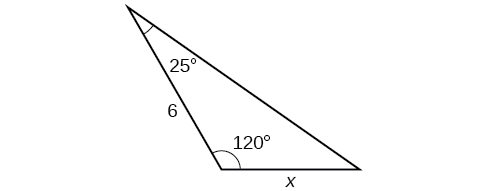33)$$12.2$$

34)35)$$16.0$$

36)For the exercises 37-,42 find the measure of angle $$x$$, if possible. Round to the nearest tenth.

37)$$29.7^{\circ}$$

38)39)$$x=76.9^{\circ}$$ or $$x=103.1^{\circ}$$

40)41) Notice that $$x$$ is an obtuse angle.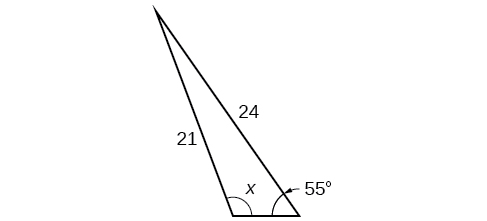$$110.6^{\circ}$$

42)For the exercises 43-49, find the area of each triangle. Round each answer to the nearest tenth.

43)$$A\approx 39.4, C\approx 47.6, BC\approx 20.7$$

44)45)$$57.1$$

46)47)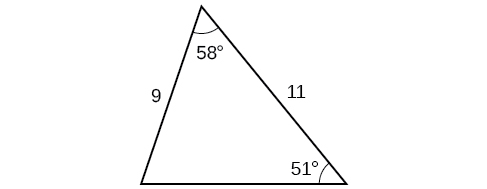$$42.0$$

48)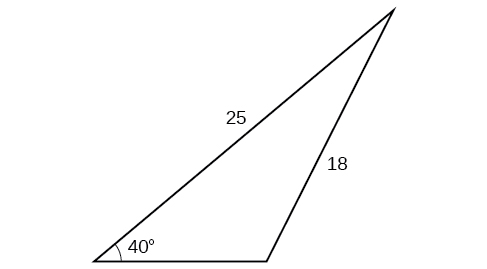49)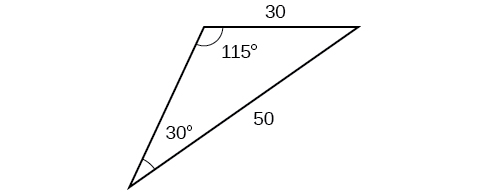$$430.2$$

### Extensions

50) Find the radius of the circle in the Figure below. Round to the nearest tenth.

51)  Find the diameter of the circle in the Figure below. Round to the nearest tenth.$$10.1$$

52) Find $$m\angle ADC$$ in the Figure below. Round to the nearest tenth.

53)  Find side $$AD$$ in the Figure below. Round to the nearest tenth.$$AD\approx 13.8$$

54) Solve both triangles in the Figure below. Round each answer to the nearest tenth.

55)  Find side $$AB$$ in the parallelogram shown below. Round to the nearest tenth.$$AB\approx 2.8$$

56) Solve the triangle in the Figure below. (Hint: Draw a perpendicular from $$H$$ to $$JK$$. Round each answer to the nearest tenth.

57)  Solve the triangle in the Figure below. (Hint: Draw a perpendicular from $$N$$ to $$LM$$. Round each answer to the nearest tenth.$$L\approx 49.7 ^{\circ} , N\approx 56.3 ^{\circ} , LN\approx 5.8$$

58) In the Figure below, $$ABCD$$ is not a parallelogram. $$\angle m$$ is obtuse. Solve both triangles. Round each answer to the nearest tenth.### Real-World Applications

59) A pole leans away from the sun at an angle of $$7^{\circ}$$ to the vertical, as shown in the Figure below. When the elevation of the sun is $$55^{\circ}$$, the pole casts a shadow $$42$$ feet long on the level ground. How long is the pole? Round the answer to the nearest tenth.$$51.4$$ ft

60) To determine how far a boat is from shore, two radar stations $$500$$ feet apart find the angles out to the boat, as shown in the Figure below. Determine the distance of the boat from station $$A$$ and the distance of the boat from shore. Round your answers to the nearest whole foot.

61) The Figure below shows a satellite orbiting Earth. The satellite passes directly over two tracking stations $$A$$ and $$B$$, which are $$69$$ miles apart. When the satellite is on one side of the two stations, the angles of elevation at $$A$$ and $$B$$ are measured to be $$86.2^{\circ}$$ and $$83.9^{\circ}$$ respectively. How far is the satellite from station $$A$$ and how high is the satellite above the ground? Round answers to the nearest whole mile.The distance from the satellite to station $$A$$ is approximately $$1716$$ miles. The satellite is approximately $$1706$$ miles above the ground.

62) A communications tower is located at the top of a steep hill, as shown in the Figure below. The angle of inclination of the hill is $$67^{\circ}$$. A guy wire is to be attached to the top of the tower and to the ground, $$165$$ meters downhill from the base of the tower. The angle formed by the guy wire and the hill is $$16^{\circ}$$. Find the length of the cable required for the guy wire to the nearest whole meter.

63) The roof of a house is at a $$20^{\circ}$$ angle. An $$8$$-foot solar panel is to be mounted on the roof and should be angled $$38^{\circ}$$ relative to the horizontal for optimal results. (See the Figure below). How long does the vertical support holding up the back of the panel need to be? Round to the nearest tenth.$$2.6$$ ft

64) Similar to an angle of elevation, an angle of depression is the acute angle formed by a horizontal line and an observer’s line of sight to an object below the horizontal. A pilot is flying over a straight highway. He determines the angles of depression to two mileposts, $$6.6$$ km apart, to be $$37^{\circ}$$ and $$44^{\circ}$$ as shown in the Figure below. Find the distance of the plane from point $$A$$ to the nearest tenth of a kilometer.

65) A pilot is flying over a straight highway. He determines the angles of depression to two mileposts, $$4.3$$ km apart, to be $$32^{\circ}$$ and $$56^{\circ}$$, as shown in the Figure below. Find the distance of the plane from point $$A$$ to the nearest tenth of a kilometer.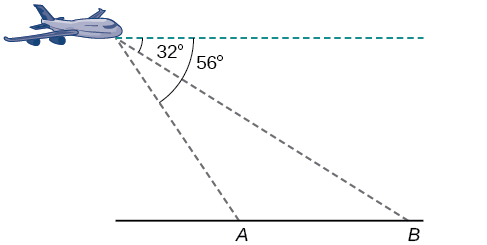$$5.6$$ km

66) In order to estimate the height of a building, two students stand at a certain distance from the building at street level. From this point, they find the angle of elevation from the street to the top of the building to be $$39^{\circ}$$. They then move $$300$$ feet closer to the building and find the angle of elevation to be $$50^{\circ}$$. Assuming that the street is level, estimate the height of the building to the nearest foot.

67) In order to estimate the height of a building, two students stand at a certain distance from the building at street level. From this point, they find the angle of elevation from the street to the top of the building to be $$35^{\circ}$$. They then move $$250$$ feet closer to the building and find the angle of elevation to be $$53^{\circ}$$. Assuming that the street is level, estimate the height of the building to the nearest foot.

$$371$$ ft

68) Points $$A$$ and $$B$$ are on opposite sides of a lake. Point $$C$$ is $$97$$ meters from $$A$$. The measure of $$\angle BAC$$ is determined to be $$101^{\circ}$$, and the measure of $$\angle ACB$$ is determined to be $$53^{\circ}$$. What is the distance from $$A$$ to $$B$$$,$rounded to the nearest whole meter?

69) A man and a woman standing $$3\dfrac{1}{2}$$ miles apart spot a hot air balloon at the same time. If the angle of elevation from the man to the balloon is $$27^{\circ}$$, and the angle of elevation from the woman to the balloon is $$41^{\circ}$$, find the altitude of the balloon to the nearest foot.

$$5936$$ ft

70) Two search teams spot a stranded climber on a mountain. The first search team is $$0.5$$ miles from the second search team, and both teams are at an altitude of $$1$$ mile. The angle of elevation from the first search team to the stranded climber is $$15^{\circ}$$. The angle of elevation from the second search team to the climber is $$22^{\circ}$$. What is the altitude of the climber? Round to the nearest tenth of a mile.

71) A street light is mounted on a pole. A $$6$$-foot-tall man is standing on the street a short distance from the pole, casting a shadow. The angle of elevation from the tip of the man’s shadow to the top of his head of $$28^{\circ}$$. A $$6$$-foot-tall woman is standing on the same street on the opposite side of the pole from the man. The angle of elevation from the tip of her shadow to the top of her head is $$28^{\circ}$$. If the man and woman are $$20$$ feet apart, how far is the street light from the tip of the shadow of each person? Round the distance to the nearest tenth of a foot.

$$24.1$$ ft

72) Three cities, $$A$$, $$B$$, and $$C$$, are located so that city $$A$$ is due east of city $$B$$. If city $$C$$ is located $$35^{\circ}$$ west of north from city $$B$$ and is $$100$$ miles from city $$A$$ and $$70$$ miles from city $$B$$, how far is city $$A$$ from city $$B$$? Round the distance to the nearest tenth of a mile.

73) Two streets meet at an $$80^{\circ}$$ angle. At the corner, a park is being built in the shape of a triangle. Find the area of the park if, along one road, the park measures $$180$$ feet, and along the other road, the park measures $$215$$ feet.

$$19,056$$ ft2

74) Brian’s house is on a corner lot. Find the area of the front yard if the edges measure $$40$$ and $$56$$ feet, as shown in the Figure below.75) The Bermuda triangle is a region of the Atlantic Ocean that connects Bermuda, Florida, and Puerto Rico. Find the area of the Bermuda triangle if the distance from Florida to Bermuda is $$1030$$ miles, the distance from Puerto Rico to Bermuda is $$980$$ miles, and the angle created by the two distances is $$62^{\circ}$$.

$$445,624$$ square miles

76) A yield sign measures $$30$$ inches on all three sides. What is the area of the sign?

77) Naomi bought a modern dining table whose top is in the shape of a triangle. Find the area of the table top if two of the sides measure $$4$$ feet and $$4.5$$ feet, and the smaller angles measure $$32^{\circ}$$ and $$42^{\circ}$$, as shown in the Figure below.$$8.65$$ ft2

## 8.2: Non-right Triangles - Law of Cosines

### Verbal

1) If you are looking for a missing side of a triangle, what do you need to know when using the Law of Cosines?

two sides and the angle opposite the missing side.

2) If you are looking for a missing angle of a triangle, what do you need to know when using the Law of Cosines?

3) Explain what $$s$$ represents in Heron’s formula.

## 8.6: Parametric Equations

### Technology

$$t$$ $$x$$ $$y$$
-1
0
1

$$t$$ $$x$$ $$y$$
1
2
3
$$t$$ $$x$$ $$y$$
1 -3 1
2 0 7
3 5 17

$$t$$ $$x$$ $$y$$
-1
0
1
2

## 8.7: Parametric Equations - Graphs

### Graphical

$$t$$ $$x$$ $$y$$
-3
-2

 $$t$$ $$x$$ $$y$$ -3 -2 -1 0 1 2

 $$t$$ $$x$$ $$y$$ -2 -1 0 1 2 3

 $$t$$ $$x$$ $$y$$ -3 -2 -1 0 1

 $$t$$ $$x$$ $$y$$ -2 -1 0 1 2

 $$t$$ $$x$$ $$y$$ -2 -1 0 1 2